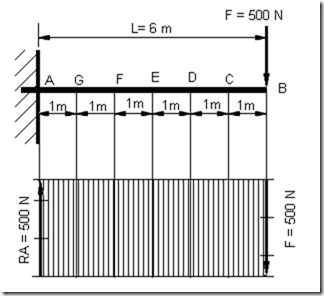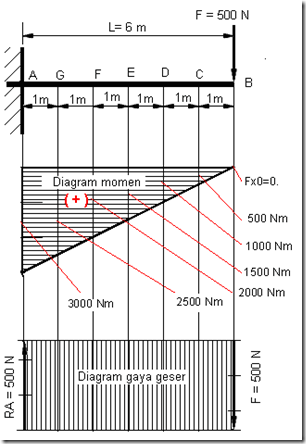Home > English > Diagram Moment and Style Slide

Diagram Moment and Style Slide

a. Diagram Moment

Moment diagram is a picture or diagram that shows the magnitude of the moment in any place and made in accordance with the scale of the moment. The scale of this moment adapted to the situation / circumstances papers widely available. for example 1cm appeal of 100 Nm, which means that every length of 1 cm show the amount of torque of 100 Nm, to distinguish the direction of moments then for the moment with the direction of the direction of clockwise is called the positive moments and vice versa moment opposite direction to clockwise called negative moment ,

The moment diagram can be seen in the following figure.Figure 6.2 Diagram of torque on the shaft

When the rod AB of length L at the end of A at the free-flops and the other end is not in the fulcrum, at the end of the F B is loaded vertically downwards then the end will arise A shear force and bending moment. The magnitude of the bending moment in accordance with the magnitude of the load F and the distance. From drawing 6.2 magnitude bending moment at each of each point is as follows:
o Moment at point B is MB = Fx0 = 0.
o Moment at point C, namely MX1 = F.X1.
o Moment at point D ie MX2 = F.X2.
o Moment at point E, namely MX3 = F.X3.
o Moment at point F is MX4 = F.X4.
o Moment at point G is MX5 = F.X5.
o The moment at point A is MX6 = F.X6. = FXL.

b. Diagram Style Slide
Shear force diagrams are also called force field latitude of images that show the amount of shear force that works in any place along the stems get shear loads. The shear force given the symbol Dx. If the shear forces unmarked ppositif upward direction and if the downward direction of the shear force is negative.
At loading on the stem AB, getting shear load of 500 N For local AB or 0 <x <6m Dx = 500 N. See the following page images,Figure 6.5 Diagram of shear

c. Combined Diagram Moment And Style Slide
Moment diagram and shear force diagrams are usually drawn in a single drawing or combined, and for ease of calculation normally used tables as shown in the following table.

Table 6. 2 Moment and Style SlideFigure 6.6 Diagram of torque and shear force

d. Stem ditumpu With Two PedestalFigure 6.10 Trunk ditumpu at both ends

A rod that has a length L at both ends ditumpu, at the end of the A ditumpu with pedestal roller and swept others ditumpu with pedestal hinge, in the middle of the rod AB is at point C within ½ L from point A burdened by force F [N] , Determine the reaction force in the foundation of the A and B reaction in pedestal, if the rod is in a state of balance."Tasikmalaya, Indonesia"